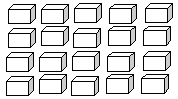Name: ___________________Date:___________________

 Email us to get an instant 20% discount on highly effective K-12 Math & English kwizNET Programs!

### Grade 2 - Mathematics8.1 Final Review Test

 Q 1: How much is 7 dimes, 2 nickels and 8 pennies?92 cents98 cents88 cents Q 2: Use the figure to find 5 times 4.212019 Q 3: 9 + 9 =018107 Q 4: Write the multiplication form for 5 + 5 + 5 + 5 + 5 + 55 X 5 = 255 + 5 = 105 X 6 = 30 Q 5: The answer in multiplication is called the _______.unknownproductdividendquotient Q 6: Is this figure symmetric?noyes Q 7: 12 - 7 =31975 Q 8: Find the difference of the two numbers shown below.Answer: Question 9: This question is available to subscribers only! Question 10: This question is available to subscribers only!